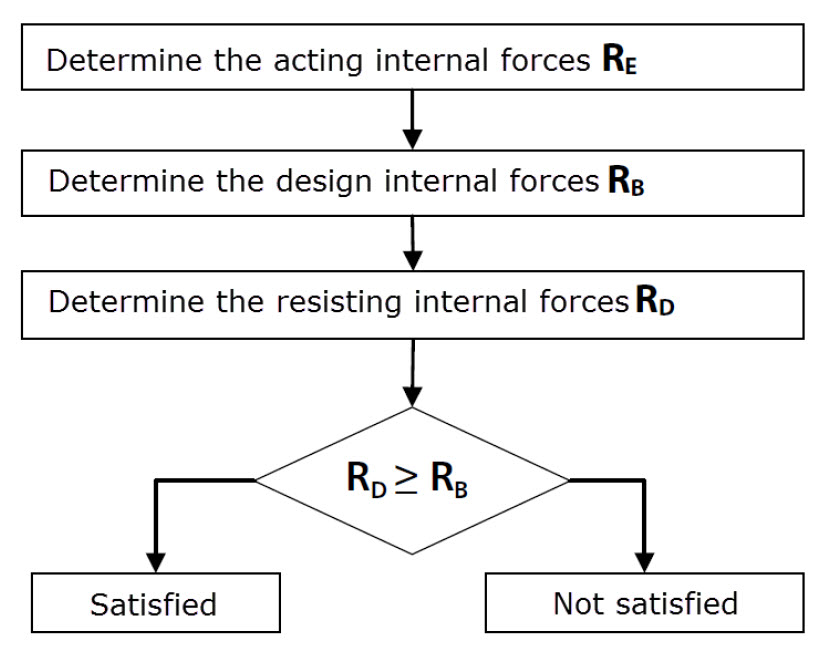# RF-CONCRETE Surfaces – Online Manual Version 5

Online manuals, introductory examples, tutorials, and other documentation.

# 2.2 Design of 1D and 2D Structural Components

To design the ultimate limit state of a one- or two-dimensional structural component consisting of reinforced concrete, it is always necessary to find a state of equilibrium between the acting internal forces and the resisting internal forces of the deformed component. However, in addition to this common feature in the ultimate limit state design of one-dimensional structural components (members) and two-dimensional components (surfaces), there is a crucial difference:

1D structural component (member)

In a member, the acting internal force is always oriented in such a way that it can be compared to the resisting internal force that is determined by the design strengths of the materials. An example of this is a member subjected to the axial compressive force N.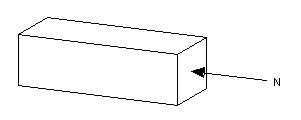Figure 2.2 Design of a member

With the dimensions of the structural component and the design value of the concrete compressive strength, it is possible to determine the resisting compressive force. If it is smaller than the acting compressive force, the required area of the compressive reinforcement can be determined by means of the existing steel strain with an allowable concrete compressive strain.

2D structural component (surface)

In a surface, the direction of the acting internal force is only in exceptional cases (trajectory reinforcement) oriented in such a way that the directly acting internal force can be set in contrast with the resisting internal force: In an orthogonally reinforced wall, for example, the directions of the two principal axial forces n1 and n2 are generally not identical to the reinforcement directions.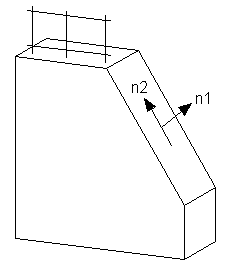Figure 2.3 Design of a wall

Hence, for the dimensioning of the mesh's reinforcement, it is not possible to use such an approach as for determining a member's reinforcement. Internal forces that run in the direction of the reinforcement mesh's layers are required in order to determine the concrete loading. These internal forces are called design internal forces.

To better understand the design internal forces, we can look at an element of a loaded reinforcement mesh. For simplicity's sake, we assume the second principal axial force n2 to be zero.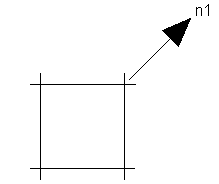Figure 2.4 Reinforcement mesh element with loading

The reinforcement mesh deforms under the given loading as follows.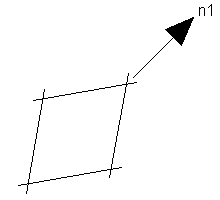Figure 2.5 Deformation of reinforcement mesh element

The size of the deformation is limited by introducing a concrete compression strut to the reinforcement mesh element.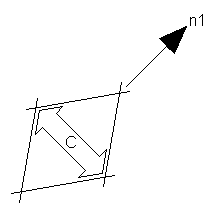Figure 2.6 Introducing a concrete compression strut

The concrete compression strut induces tensile forces in the reinforcement.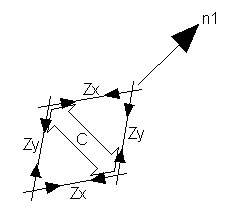Figure 2.7 Tensile forces in the reinforcement

These tensile forces in the reinforcement and the compressive force in the concrete represent the design internal forces.

Once the design internal forces are found, the design can proceed like the design of a one-dimensional structural component.

Thus, the main feature for the design of two-dimensional structural components is the transformation of the acting internal forces (principal internal forces) into design internal forces whose direction allows for dimensioning the reinforcement and checking the concrete's load-bearing capacity.

The following graphic illustrates the main difference between the design of one-dimensional and two-dimensional structural components.

One-dimensional structural component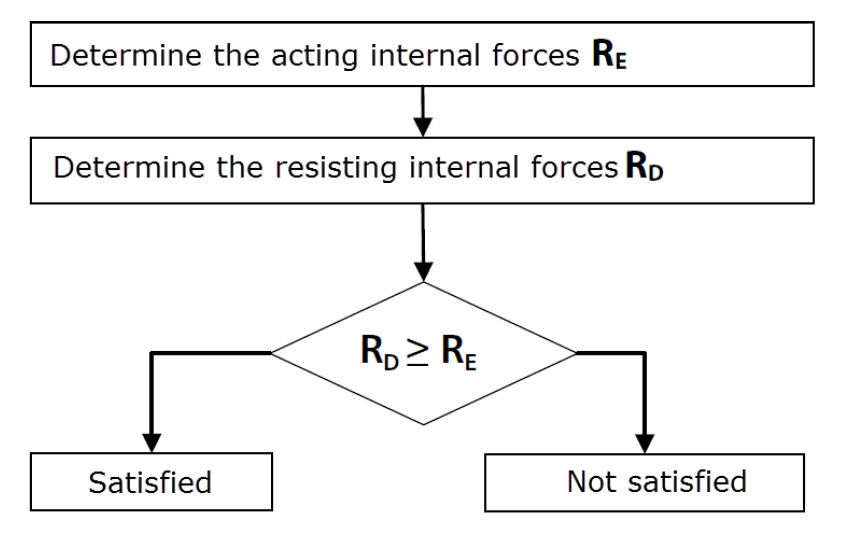Two-dimensional structural component